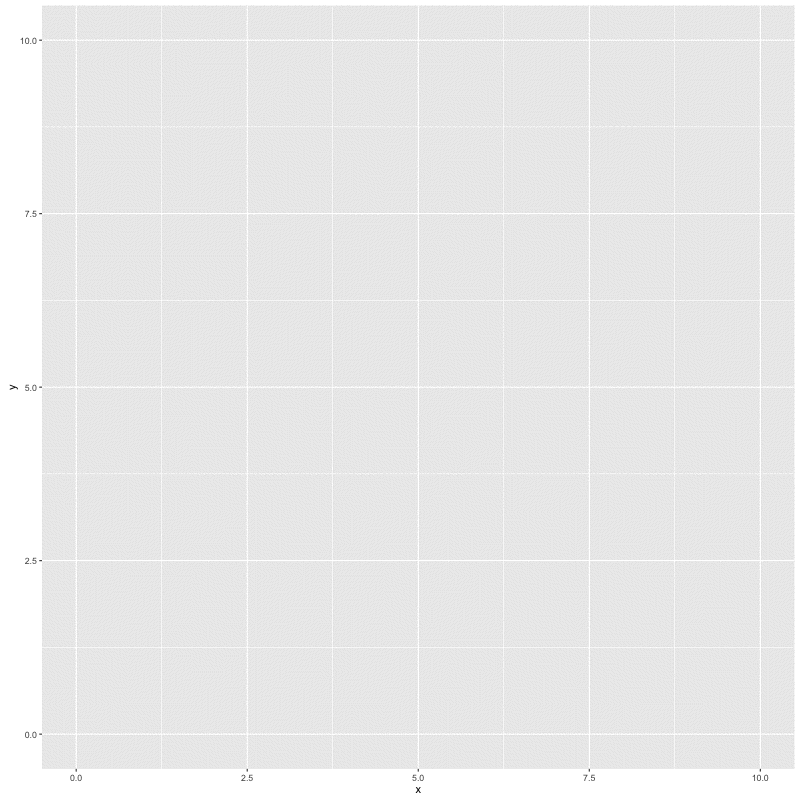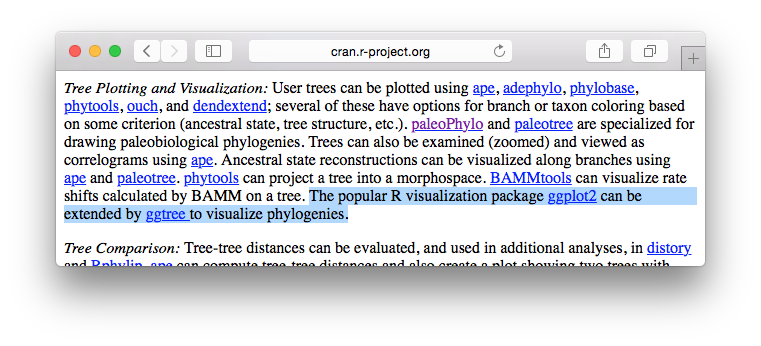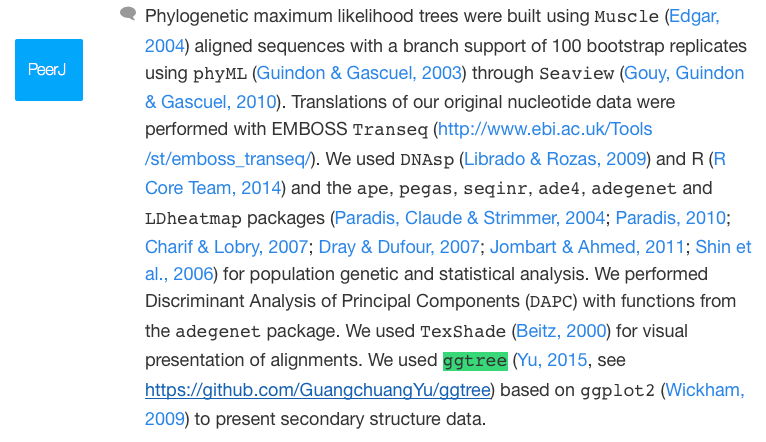I implemented a function, subview, in ggtree that make it easy to embed a subplot in ggplot.

An example is shown below:

``````library(ggplot2)
library(ggtree)

dd <- data.frame(x=LETTERS[1:3], y=1:3)
pie <- ggplot(dd, aes(x=1, y, fill=x)) +
geom_bar(stat="identity", width=1) +
coord_polar(theta="y") + theme_tree() +
xlab(NULL) + ylab(NULL) +
theme_transparent()

x <- sample(2:9)
y <- sample(2:9)
width <- sample(seq(0.05, 0.15, length.out=length(x)))
height <- width

p <- ggplot(data=data.frame(x=c(0, 10), y=c(0, 10)), aes(x, y))+geom_blank()
print(p)
for (i in seq_along(x)) {
p %<>% subview(pie, x[i], y[i], width[i], height[i])
print(p)
}
``````With this function, we can plot a specific clade and add a subplot of the whole topology; we can also add relative statistic graph above the tree or to a specific node. It’s not specific to phylogenetic tree, it works with all ggplot objects as demonstrated in the example.

PS: ggtree is now presented in CRAN Task View: Phylogenetics.AND get its first citation.## Citation

G Yu, DK Smith, H Zhu, Y Guan, TTY Lam*. ggtree: an R package for visualization and annotation of phylogenetic trees with their covariates and other associated data. Methods in Ecology and Evolution. `doi:10.1111/2041-210X.12628`.